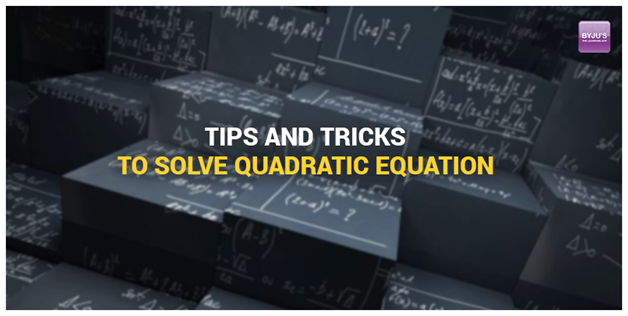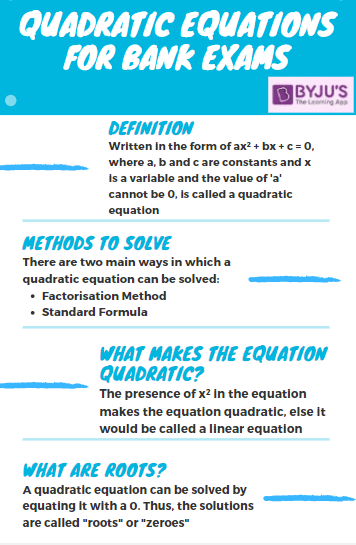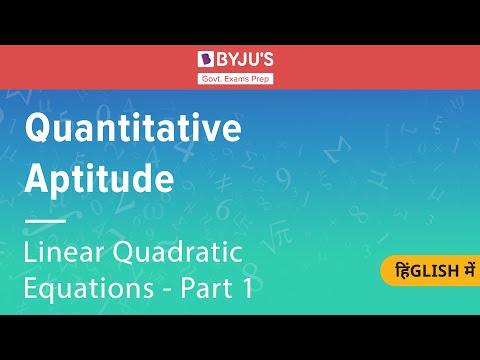# Top Tips To Solve Quadratic Equations In Bank Exams

Quadratic equation is one of the most complex and tricky parts of Quantitative Aptitude in the Bank PO and clerk exams where candidates generally make silly mistakes if they do not focus during their bank exam preparation.

As a result, there are some tricks and strategies which are essential to solve a quadratic equation. Bank exams require accuracy in the allotted time during the main exam so shortcut tricks are important for an aspirant to quickly solve a particular bank exam question.

To get the detailed syllabus for the quantitative aptitude section for various bank exams, candidates can visit the linked article.

The numerical ability section is where the candidates can score well by practising well within a given time using more tricks during their bank exam preparation.## Quadratic Equation – Basic Concept

The quadratic equations are one of the most commonly asked questions in the bank examinations. Mostly questions from this topic are asked in the form of inequalities in the first phase of the exam and also helps in various other word problems which can be solved by forming equations.Candidates who are preparing for the upcoming bank exams 2023 must dedicate quality time in understanding and solving more and more quadratic equation-based questions so that they develop a command over solving such questions and do not spend too much time with questions from this topic.

Given below are a few other links which will help you prepare for the quantitative aptitude section and score more in the bank exams 2023:

For candidates who are not much familiar with the concept of quadratic equation and the type of questions which may be asked from this topic can refer to the video given below to get the best assistance:### Weightage of Quadratic Equation in Bank Exams

 Priority Nature of expected questions Weightage 1 Maintaining accuracy, problems that can be solved easily, easy to manage time, scoring in nature 0-5

To explore various Govt Exams, check at the linked article.

### Stepwise Tips to practice a Quadratic Equation

• Firstly the candidate needs to note down twenty sums related to the quadratic equation on a page.
• Then ten sums are to be solved using basic formula.
• To solve these sums using a stopwatch will help in time management.
• The next step is to evaluate the time taken and analyse the performance.
• The remaining ten questions are to be solved next by applying the shortcut tricks.
• The time taken should be noted carefully.
• This will surely have a difference from the time taken while solving the first ten questions.
• Practice is the key to master this topic of the Quantitative Aptitude section.

To explore the SBI Clerk Prelims Quantitative Syllabus, check at the linked article.

The basic equation of quadratic equation is

$$\begin{array}{l}ax^{2}+ bx+c =0\end{array}$$
where this equation is equal to zero and a,b,c are constants. The quadratic equation only holds the power of x where x is also known as a non-negative integer.

For example:

$$\begin{array}{l}6x^{2} + 11x +3= 0\end{array}$$

Solution: In this equation 6 is the coefficient of

$$\begin{array}{l}x^{2}\end{array}$$

11 is the coefficient of x and 3 is constant. The easiest way is to solve this through the middle term break method.

First step:  To multiply

$$\begin{array}{l}(+6)\times (+3)\end{array}$$
= +18

Second step: +18 is to be broken into two parts in such a way that their addition results in the middle term that is 11 where

$$\begin{array}{l}9 + 2 = 11\end{array}$$
and product of these factors are 18.

Third step: To change the sign of the factors where +9 = -9 and +2 =-2 and this has to be divided by coefficient of

$$\begin{array}{l}x^{2}\end{array}$$
. Therefore,
$$\begin{array}{l}-9/6 = -3/2\end{array}$$
and
$$\begin{array}{l}-2/6 = -1/3\end{array}$$
.

To learn how to Prepare Quantitative Aptitude For Bank Exams, check at the linked article.

 To solve more questions based on quadratic equations, candidates must solve more and more Free Mock Tests Online. Also, refer to the links given below to ace the upcoming Bank exams:

### Top 3 Tips to solve Quadratic Equations in Bank Exams

The major three ways to solve the problems on the Quadratic Equation are as follows:

1. To factor the Quadratic Equation- Here all the same terms are to be combined and to the one side of the equation in such a way that there remains nothing on the other side. Once it has no remaining terms we can write zero.
For example:
$$\begin{array}{l}3x^{2} – 8x -4 = 3x + x^{2}\end{array}$$
$$\begin{array}{l}4x^{2} – 11x – 4=0\end{array}$$
The second step is to factor the equation so that there is a set each through the middle term break method. The last part is to separate each factor set to zero.
For example:
$$\begin{array}{l}(3x+1)(x-4)=0\end{array}$$
2. To use the formula- The sums can be solved through the formula
$$\begin{array}{l}\frac{-b\pm \sqrt{b^{2}-4ac}}{2a}\end{array}$$
and solve putting the equations in this.
3. To complete the square- This process is bit complex while the other two are easy to solve.

To learn Tips To Solve Important Maths Questions of SBI PO Exam, check at the linked article.

We hope the above-mentioned shortcut tricks and strategies will help the candidates during their bank exam preparation for Quadratic Equations. All the candidate needs to do is practice with determination and hard work to crack the bank exams successfully.

A few other quantitative aptitude related links have been given below for your assistance:

### Quadratic Equation Sample Questions for Bank Exams

Given below are a few sample questions which will help candidates understand the concept of quadratic equations even better and get habitual of solving such questions within a shorter time span.

Directions (Q1 – Q4): Solve the following quadratic equations and choose the correct answer from the options given below:

Q 1. Equation I: x2 – 28x + 195 = 0
Equation II: y2 – 30y + 216 = 0

1. x>y
2. y>x
3. x=y, or no relation
4. x≤y
5. x≥y

Answer: (3) x=y, or no relation

Solution:

Equation I: x2 – 28x + 195 = 0

⇒ x2 – 13x – 15x + 195 = 0

⇒ x (x-13) – 15 (x-13)

⇒ x = 13/ 15

Equation II: y2 – 30y + 216 = 0

⇒ y2 – 12y – 18y + 216 = 0

⇒y (y-12) – 18 (y-12) = 0

⇒ y = 12/ 18

Thus, no relation can be found between the x and y

Q 2. Equation I: (y – 18)² = 0

Equation II: x² = 324

1. x>y
2. y>x
3. x=y, or no relation
4. x≤y
5. x≥y

Solution:

Equation I: (y – 18)² = 0

⇒ Using the formula, (y – 18)² = y2 +(18)2 – (2×18×y)

⇒ y2 + 324 – 36y = 0

⇒ y2 – 36y + 324 = 0

⇒ y2 – 18y -18y + 324 = 0

⇒ y (y-18) – 18 (y-18) = 0

⇒ y = +18/ +18

Equation II: x² = 324

x = -18/ +18

Q 3. Equation I: 3x² + 20x + 33 = 0

Equation II: 2y² – 13y + 21 = 0

1. x>y
2. y>x
3. x=y, or no relation
4. x≤y
5. x≥y

Solution:

Equation I: 3x² + 20x + 33 = 0

3x² + 20x + 33 = 0

⇒ 3x² + 9x + 11x + 33 = 0

⇒ 3x (x+3) + 11 (x+3) = 0

⇒ x = -3/ -3.67

Equation II:

2y² – 13y + 21 = 0

⇒ 2y² – 6y -7y + 21 = 0

⇒ 2y (y-3) – 7 (y-3)

⇒ y = 3/ 3.5

Q 4. Equation I: p² – 27p + 180 = 0

Equation II: q² + 20q + 91 = 0

1. p>q
2. q>p
3. p=q, or no relation
4. p≤q
5. p≥q

Solution:

Equation I: p² – 27p + 180 = 0

⇒ p² – 15p – 12p + 180 = 0

⇒ p (p-15) – 12 (p-15) = 0

⇒ p = 12/ 15

Equation II: q² + 20q + 91 = 0

⇒ q² + 13q + 7q + 91 = 0

⇒ q (q+13) + 7 (q+13) = 0

⇒ q = -13/ -7

Candidates must solve more such questions based on quadratic equation to completely understand how to solve the quadratic equations and find the value of the variables.